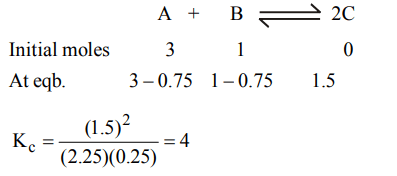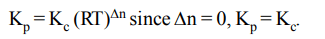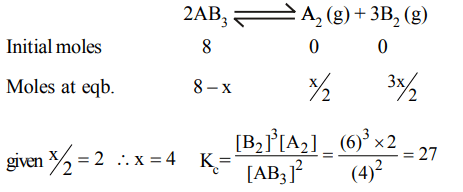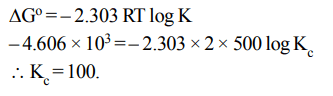## Chemical Equilibrium Questions and Answers Part-7

1. When 3 mole of reactant A and one mole of the reactant B are mixed in a vessel of volume 1 litre, the following reaction takes place $A\left(g\right)+B\left(g\right)\rightleftharpoons 2C\left(g\right)$     If 1.5 mole of C is formed at equilibrium, the equilibrium constant $\left(K_{C}\right)$ of the reaction is
a) 0.12
b) 0.50
c) 0.25
d) 4.00

Explanation:2. Kc for the reaction $N_{2}\left(g\right)+O_{2}\left(g\right)\rightleftharpoons 2NO\left(g\right)$      at 300 K is $4.0 × 10^{-6}$ . $K_{P}$ for the above reaction will be $\left(R=2 cal mol^{-1}K^{-1}\right)$
a) $2.4 × 10^{-3}$
b) $4 × 10^{-6}$
c) $4 × 10^{-6}\left(RT\right)^{2}$
d) $16 × 10^{-12}$

Explanation:3. Which of the following equilibria will shift to right side on increasing the temperature?
a) $CO \left(g\right)+H_{2}O\left(g\right)\rightleftharpoons CO_{2} \left(g\right)+H_{2}\left(g\right)$
b) $2SO_{2} \left(g\right)+O_{2}\left(g\right)\rightleftharpoons 2SO_{3}\left(g\right)$
c) $H_{2}O\left(g\right)\rightleftharpoons H_{2}\left(g\right)+1\diagup2 O_{2} \left(g\right)$
d) $4HCI\left(g\right)+O_{2}\left(g\right)\rightleftharpoons {2}H_{2}O\left(g\right)+2CI_{2} \left(g\right)$

Explanation: Reaction (c) is endothermic. Electrolysis or decomposition of H2O is endothermic in nature.

4. 1 mole of $N_{2}$ and 2 moles of $H_{2}$ are allowed to react in a $1 dm^{3}$ vessel. At equilibrium 0.8 mole of $NH_{3}$ is formed. The concentration of $H_{2}$ in the vessel is
a) 0.6 mole
b) 0.8 mole
c) 0.2 mole
d) 0.4 mole

Explanation:5. The equilibrium $SO_{2}Cl_{2}\left(g\right)\rightleftharpoons SO_{2}\left(g\right) + Cl_{2}\left(g\right)$      is attained at 25°C in a closed container and an inert gas, helium is introduced. Which of the following statement is correct
a) More chlorine is formed
b) Concentration of $SO_{2}$ is reduced
c) More $SO_{2}Cl_{2}$  is formed
d) Concentration of $SO_{2}Cl_{2},SO_{2} and Cl_{2}$    do not change

Explanation: No change in concentration at constant volume only pressure is increased when some inert gas is introduced.

6. For which reaction high pressure and high temperature is helpful in obtaining a high equilibrium yield
a) $2NF_{3}\left(g\right)\rightleftharpoons N_{2}\left(g\right)+3F_{2}\left(g\right) – 54.40 kcal$
b) $N_{2}\left(g\right)+3H_{2}\left(g\right) \rightleftharpoons 2NH_{3}\left(g\right) + 22.08 kcal$
c) $Cl_{2}\left(g\right)+2O_{2}\left(g\right) \rightleftharpoons 2ClO_{2}\left(g\right) – 49.40 kcal$
d) $2Cl_{2}O_{7}\left(g\right) \rightleftharpoons 2Cl_{2}\left(g\right) +7O_{2}\left(g\right)+ 126.8 kcal$

Explanation: $Cl_{2}\left(g\right)+2O_{2}\left(g\right) \rightleftharpoons 2ClO_{2}\left(g\right) – 49.40 kcal$

7. For the reversible reaction $N_{2}\left(g\right)+3H_{2}\left(g\right) \rightleftharpoons2NH_{3}\left(g\right)$      at 500°C, the value of $K_{P}$ is $1.44 × 10^{-5}$   when partial pressure is measured in atmospheres. The corresponding value of $K_{C}$ with concentration in mole litre-1, is
a) $1.44 × 10^{-5}/ (0.082 × 500)^{-2}$
b) $1.44 × 10^{-5}/ (8.314 × 773)^{-2}$
c) $1.44 × 10^{-5}/ (0.082 × 773)^{2}$
d) $1.44 × 10^{-5}/ (0.082 × 773)^{-2}$

Explanation:8. A 1 M solution of glucose reaches dissociation equilibrium according to the equation given below $6HCHO\rightleftharpoons C_{6}H_{12}O_{6}$     . What is the concentration of HCHO at equilibrium if equilibrium constant is $6 × 10^{22}$
a) $1.6 × 10^{-8}M$
b) $3.2 × 10^{-6}M$
c) $3.2 × 10^{-4}M$
d) $1.6 × 10^{-4}M$

Explanation:9. 8 mole of a gas $AB_{3}$ are introduced into a 1.0 dm3 vessel. It dissociates as,$2AB_{3}\left(g\right)\rightleftharpoons A_{2}\left(g\right) + 3B_{2}\left(g\right)$      . At equilibrium, 2 mole of $A_{2}$ are found to be present. The equilibrium constant of reaction is ........ in $mol^{2}L^{-2}$
a) 2
b) 3
c) 27
d) 3610. $\triangle G^{\circ}$ for the reaction $X + Y\rightleftharpoons Z$   is –ve 4.606 kcal. The equilibrium constant for the reaction at 227°C is.